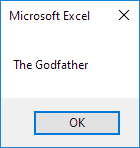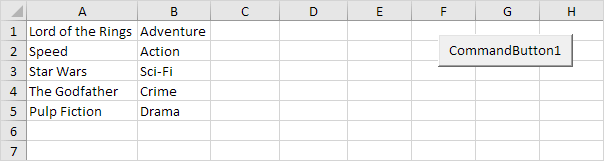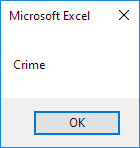# Array in Excel VBA

An array is a group of variables. In Excel VBA, you can refer to a specific variable (element) of an array by using the array name and the index number.

### One-dimensional Array

To create a one-dimensional array, execute the following steps.

Place a command button on your worksheet and add the following code lines:

Dim Films(1 To 5) As String

Films(1) = "Lord of the Rings"
Films(2) = "Speed"
Films(3) = "Star Wars"
Films(4) = "The Godfather"
Films(5) = "Pulp Fiction"

MsgBox Films(4)

Result when you click the command button on the sheet:Explanation: the first code line declares a String array with name Films. The array consists of five elements. Next, we initialize each element of the array. Finally, we display the fourth element using a MsgBox.

### Two-dimensional Array

To create a two-dimensional array, execute the following steps. This time we are going to read the names from the sheet.Place a command button on your worksheet and add the following code lines:

Dim Films(1 To 5, 1 To 2) As String
Dim i As Integer, j As Integer

For i = 1 To 5
For j = 1 To 2
Films(i, j) = Cells(i, j).Value
Next j
Next i

MsgBox Films(4, 2)

Result when you click the command button on the sheet:Explanation: the first code line declares a String array with name Films. The array has two dimensions. It consists of 5 rows and 2 columns. Tip: rows go first, then columns. The other two variables of type Integer are used for the Double Loop to initialize each element of the array. Finally, we display the element at the intersection of row 4 and column 2.

Go to Next Chapter: Function and Sub